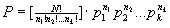（2001年2月下旬）二项分布

如果掷两次硬币，根据独立事件的概率乘法定理那么两次都是正面（反面）向上的概率是0.5×0.5=0.25。另外第一个是正第二个是反的出现概率也是0.5×0.5=0.25。同理第一个反第二个正的出现概率也是0.5×0.5=0.25。于是一正一反的概率是前面两个情况的和，即0.25+0.25=2×0.25=0.5 。它们的合计值仍然是1。列成表就是：

 两个正面的概率 一正一反的概率 两个反面的概率 0.25 2×0.25=0.5 0.25

注意到代数学中

(a+b)2=a2+2ab+b2,

而在a=0.5，b=0.5时，有

12=(0.5+0.5)2=0.25+2×0.5×0.5+0.25=1

这说明掷两次硬币的各个结局的出现概率可以通过对二项式的平方展开而得到。顺此，对于掷n次硬币的各种结局的出现概率也可以通过对二项式的n次方的展开而得到。

例如N=3时,有（注意0.5×0.5×0.5=0.125）

13=(0.5+0.5)3=0.125+3×0.125+3×0.125+0.125=

0.125+0.375+0.375+0.125=1

上式4项中的4个概率值0.1250.3750.3750.125分别对于3正、21反、12反和3反这四种结局。

(a+b)n=an+nan-1b++[n!/m!(n-m)!](an-mbm)+bn

ab分别等于0.5代入上式我们就得到n+1项，以其通项而论，它就代表了有n-m个正面m个反面的事件的出现概率。即这种类型的问题（如掷多次硬币）的概率分布恰好可以用二项式展开的牛顿公式表示。而这也就是为什么把这种概率分布类型称为二项分布的原因。

P=[n!/m!(n-m)!][pn-m(1-p)m]

P=[n!/m!(n-m)!][pm(1-p)n-m]多项分布(p1+ p2+…+ pk )N =1N=1

[ N!/(n1!n2!…nk!)](1/k)N=1

n1+n2+…nk=N

[N!/(n1!n2!…nk!)]1/kN

----12章结束，回本章首，去12章----